Home | Mathematics | * Sage | * Exploring Mathematics with Sage 02. Installation and Testing 03. Learning Sage 04. Trapezoidal Storage Tanks 05. Cylindrical Storage Tanks 06. Differential Equations 07. Future Value 08. Tuned Circuits 09. Fourier Methods 10. Polynomial Regression 11. Terminal VelocityShare This Page
Learning Sage

The first steps

P. Lutus Message Page

(double-click any word to see its definition)

Orientation
To navigate this multi-page article:

Use the drop-down lists and arrow icons located at the top and bottom of each page.

First, if you have come here from a search engine and you don't have Sage installed yet, visit this page, install Sage, then come back.

To best experience this tutorial, you should have access to a Sage notebook session. It's best to run a local copy of Sage in server mode (accessible through a browser) (this is all explained here).

Browser/Server Setup

The optimal setup to follow this tutorial is to open two Firefox browser tabs — one showing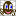this page, the other showing aSage notebook session. If you have installed Sage according to these instructions and the Sage Web server is running, then clicking here should open aSage browser tab (if you are running Linux).

• If you clicked the above link and nothing happened, this means theSage browser tab is already open — just click theSage tab in your browser.
• If you clicked the link and got an error message, this can mean:
• You haven't started the Sage server yet, or
• You're running Windows and you need to enter the address of the Sage server manually — it should look something like this: http://nnn.nnn.nnn.nnn, with random decimal digits for the 'n's.
• All these issues are explained here.

Simplicity

This tutorial is designed to minimize reader effort so the topics being discussed can take center stage. In most cases an example will be presented like this:

User entries look like this (and can be copied into a Sage worksheet)
Sage replies look like this

Although many of the following examples are static and self-explanatory, the reader is encouraged to copy the contents of the Sage entries fromthis page, switch to theSage browser tab, and paste them into an empty worksheet cell to see them in action. Do it this way:

• Clickthis page's browser tab.
• Locate an example Sage entry you want to try out:
var('a b c');q = solve(a*x^2+b*x+c==0,x);show(q)
• Drag the mouse cursor across the example text so it looks like this:
var('a b c');q = solve(a*x^2+b*x+c==0,x);show(q)
• Select the browser menu option "Edit ... Copy" or press Ctrl+C.
• Click theSage notebook browser tab.
• Click in an empty worksheet cell.
• Select the browser menu option "Edit ... Paste" or press Ctrl+V.
• To make Sage process the cell's contents, press Shift-Enter.
• After all the "ooohs" and "aahs" have died down, clickthis page's browser tab and choose another example.
Basic Math

Sage can help you do many kinds of mathematics, from the simplest to the kind used in cutting-edge research. Sage can manipulate complex equations in symbolic form or provide numeric results. Sage can draw graphs in two and three dimensions, and will let you spin its 3D graphs around for different views. Sage is a very powerful and useful tool — to me, a program like Sage alone justifies having a personal computer.

Order of Operations

Let's get started. Here's a simple math problem (my comments appear in italics):

5 + 6 * 7 + 8
Remember to press Shift-Enter to make Sage process your entry.
55

Okay. But let's check this result — maybe Sage doesn't know how to do arithmetic. Let's see — 5 plus 6 is 11, 7 plus 8 is 15, and finally, 11 times 15 is 165. Whoa — how did Sage arrive at its result? Is it broken?

Actually Sage's result is perfectly logical if we know about the mathematical Order of Operations. Without any special guidance, mathematical operations are carried out in this order:

1. Exponents and Roots, then
2. Multiplication and Division, then
So without any special intervention, Sage would proceed like this:
1. 6 * 7 = 42 (multiplication comes before addition)
2. 5 + 8 + 42 = 55

When performing nontrivial mathematics, it's very important to know about the order of operations, otherwise intuition may lead us to some bad results. But we don't have to accept the default order of operations — with a few extra keystrokes we can get any order we want. So how can we make the additions come before the multiplication? Here's how:

(5 + 6) * (7 + 8)
165

See the parentheses? In mathematics, operations inside parentheses are performed before operations outside parentheses. So the computer adds 5 + 6, then adds 7 + 8, then multiplies the two results together. This parenthesis-grouping idea is very important to understand — it's possible to force any order of operations one needs to have. And multiple levels of parentheses are permitted, with the same rules — the innermost parentheses are processed first, followed in sequence by the outer groupings.

One more comment about parentheses in Sage. If a user entry has "unbalanced" parentheses (meaning the number of '(' and ')' symbols is unequal), Sage won't tell you — instead, it will produce a very general, sometimes uninformative error message. Example:

(5 + 6) * (7 + 8
line 4
(_sage_const_4 + _sage_const_5 ) * (_sage_const_6 + _sage_const_7

^
SyntaxError: invalid syntax

In such cases, users need to figure out what "invalid syntax" means (and it doesn't always mean the same thing).

Exponents and Primes

Let's raise a number to a power:

2^16
65536

So two raised to the 16th power equals 65536. How about a bigger exponent?

2^65536
(a gigantic number with 19,729 digits)

Wow! Sage didn't even pause — it didn't have to shift gears, or whine about its salary, or anything. It's not even out of breath. It knows how to handle huge numbers.

Sidebar: How do I know the number had 19,729 digits? Well, first I got this result in the next-to-dumbest way (the dumbest way would be to count the digits):
• Turn the number into a string.
• Get the string's length.

Really brainless. But a while later my blood-sugar levels came back up and I remembered something — the number of digits in a given numerical base b (nb) can be gotten from the number of digits in base a (na) like this: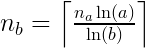(The funny-looking brackets mean "round up to the nearest integer".) As it happens, the number of digits in a binary number is equal to its power of 2, its exponent. So:= 19,729

Factoring and Functions

Okay, I'm thinking about the gigantic result we got by raising 2 to the 65,536th power, and it occurs to me that it should be divisible by two over and over again, until it equals one, without ever creating a remainder. Let's test this idea — there's a Sage function (see the "function) sidebar on this page) called "factor(x)" that can tell us:

factor(2^65536)
2^65536

Hmm. This may seem to be an obvious result, but it says the prime factors of 265536 consist of the number 2 (the only even prime number) multiplied by itself 65535 times, and "2^65536" is just a shorthand way to express the idea (otherwise we would see "2 * 2 * 2 * 2 ..." a total of 65536 times).

Moving on, I would have the reader compute the more interesting "factor(2^65536-1)", except this operation may cause your computer to become dormant for a week as it struggles to produce the result, and I am not a naturally cruel person (the difficulty of factoring large integers is the cornerstone on which modern cryptography is built). So instead I'll choose a more manageable number:

factor(2^256-1)
3 * 5 * 17 * 257 * 641 * 65537 * 274177 * 6700417 * 67280421310721 * 59649589127497217 * 5704689200685129054721

This result is a list of the prime factors of 2256-1. Many of my readers already know this, but each integer greater than 1 is either a prime number (divisible only by itself and 1) or a composite number (the product of a unique set of prime factors). This, by the way, is called the Fundamental Theorem of Arithmetic.

But are the numbers in the above result really prime? Let's find out:

is_prime(257)
True
is_prime(6700417)
True

A true mathematician (which I am not) would either think of a way to test all the numbers in the result, or would prove on first principles that they must all be prime.

What happens if the number given to "factor(x)" is prime?

factor(257)
257

So "factor(x)" is perfectly consistent — it always produces the prime factors of a given argument. Because 257 is prime, its only prime factor is itself (by definition, the number 1 is not prime).

Diagonal Thinking

I want to know how far it is from one corner of a room to the corner diagonally opposite. There are some exotic ways to compute this distance, but the simplest is based on the Pythagorean Theorem. Let's say I know the room is rectangular and is 17 by 22 feet. I know the diagonal distance is equal to the square root of the sum of the squares of the two measurements (or d = sqrt(x^2+y^2). Here's a crude effort to compute the distance:

sqrt(17^2+22^2)
sqrt(773)

What? Is Sage being deliberately obtuse? Yes, I know that 17^2+22^2 = 773, but couldn't Sage drop the other shoe and take the square root? The reason Sage didn't automatically provide a numerical result has to do with its reluctance to leave the domain of integers (according to a saying by Kronecker: "God created the integers, all the rest is the work of man") and sqrt(773) can't be represented as either an integer or a rational number. To force Sage to produce a numerical result, do this:

N(sqrt(17^2+22^2))
27.8028775489157
The function "N(x)" forces a numerical result if possible (and it's not always possible). Here's something interesting about the function "N(x)":
N(sqrt(17^2+22^2),200)
27.802877548915687730654057189532632595638578197646422961214

The second argument to "N(x)" specifies the number of binary bits in the result. This means function "N(x)" is actually defined as "N(x,b)" but the "b" is optional. Here's another "N(x,b)" example:

N(pi,1000)
```3.1415926535897932384626433832795028841971693993751058209749445923078164\
062862089986280348253421170679821480865132823066470938446095505822317253\
594081284811174502841027019385211055596446229489549303819644288109756659\
334461284756482337867831652712019091456485669234603486104543266482133936\
0726024914127
```

1000 binary bits (about 300 decimal digits) of Pi — isn't that cool? To specify 1000 decimal digits instead of binary bits, do it this way:

N(pi,digits=1000)
```3.1415926535897932384626433832795028841971693993751058209749445923078164\
062862089986280348253421170679821480865132823066470938446095505822317253\
594081284811174502841027019385211055596446229489549303819644288109756659\
334461284756482337867831652712019091456485669234603486104543266482133936\
072602491412737245870066063155881748815209209628292540917153643678925903\
600113305305488204665213841469519415116094330572703657595919530921861173\
819326117931051185480744623799627495673518857527248912279381830119491298\
336733624406566430860213949463952247371907021798609437027705392171762931\
767523846748184676694051320005681271452635608277857713427577896091736371\
787214684409012249534301465495853710507922796892589235420199561121290219\
608640344181598136297747713099605187072113499999983729780499510597317328\
160963185950244594553469083026425223082533446850352619311881710100031378\
387528865875332083814206171776691473035982534904287554687311595628638823\
53787593751957781857780532171226806613001927876611195909216420199
```

But let's not get distracted — who knows where this might lead? We have a room's diagonal to measure.

Writing Functions

Wouldn't it be nice to have a function that knows how to convert room measurements into diagonal distances? If we had such a function, we wouldn't have to enter the specific mathematical operations or the N(x) conversion each time. As it turns out, Sage will happily accept custom function definitions. Here's how to write a diagonal function:

def diagonal(x,y): return N(sqrt(x^2+y^2))
diagonal(17,22)
27.8028775489157

This example shows that a Sage function declaration begins with "def", moves on to a description (how it looks to the world) and then provides a definition (this is just one kind of function declaration, there are others to be explained later).

It is very common for Sage worksheets, once they get beyond a certain complexity level, to contain function declarations — they clarify what's going on, reduce the amount of typing required, and in the long run help us understand the process.

Here's a way to improve our diagonal function — let's say we want to accept three dimensions instead of two (in this example, that would mean the diagonal distance from one corner at the floor of the room to an opposite point at the ceiling). But the third dimension entry should be optional — the function should work for two dimensions just as before. Here's the new declaration:

def diagonal(x,y,z = 0): return N(sqrt(x^2+y^2+z^2))
diagonal(17,22)
27.8028775489157
diagonal(17,22,8)
28.9309522829789

This new function will accept a third argument, but the argument is optional — it won't prevent the function from working with two arguments. The new declaration has a "z" value that defaults to 0 (z = 0) if the user doesn't provide a different value. The second example above gives us the total three-dimensional diagonal distance (across the room and from floor to ceiling) inside a 17 x 22 foot room with an 8-foot ceiling.

Graphing

Sage can produce many kinds of two- and three-dimensional graphs, including some that accept user interactions. Let's start with a simple 2D graph:

plot(sin(x*8)^2 * e^-(x*x),x,-2,2)Now let's fill the region between the zero line and the function's curve:

plot(sin(x*8)^2 * e^-(x*x),x,-2,2,fill=True,fillcolor='blue')Now let's plot two graphs, overlaid on each other:

a = plot(sin(x*8)^2 * e^-(x*x),x,-2,2,fill=True,fillcolor='blue',fillalpha=1)
b = plot(e^-(x*x),x,-2,2,fill=True,fillcolor='yellow')
show(b+a)Accessible Help

At this point the reader may want to read more about exotic options like "fillalpha" and what they do. It turns out Sage a has very slick help system that responds to a simple user action. It works like this:

plot? [Shift-Enter]
(a window opens that describes options for "plot")

Another very slick help feature is to type the name of a word Sage knows, add ".", then press Tab to see a list of the word's functions:

plot.[Tab]
(A not-very-interesting list of functions)

Let's try this trick with a more interesting Sage word:

pi.[Tab]
(A huge list of properties and functions)

Wow. This makes me wonder what type of object "pi" is in Sage's universe:

type(pi)
<type 'sage.symbolic.expression.Expression'>

As it turns out, Sage symbolic expressions represent a very large, comprehensive class with many options and functions. Obviously not all the properties listed with "pi.[Tab]" apply to each particular member of the class.

Interactive Graph

Now we'll draw a graph that invites user interaction. This requires a somewhat larger cell content — copy and paste this multiline cell entry into a single Sage worksheet cell:

```f(x) = 5*x - x^2/8 + x^3/1200
df(x) = diff(f(x),x)
max = 100
p = plot(f(x),x,0,max)
lblp = text("\$y = " + latex(f(x)) + "\$",[40,100],fontsize=14)
lbldp = text("\$y' = " + latex(df(x)) + "\$",[40,88],fontsize=14,rgbcolor='#006000')
@interact
def _(argx=(0..max)):
dp = plot(f(argx) + (df(argx)*(x-argx)),x,0,max,color="#006000")
xp = point((argx,f(argx)),rgbcolor='#800000')
show(p+lblp+lbldp+dp+xp,ymin=0,ymax=max,axes_labels=['x','y'],fontsize=12)
```
Note: when you paste this cell's content, don't try to "fix" the indentation of certain lines — it's critical to how the cell functions.Those not pasting these examples into Sage are missing out — the above graphic image doesn't show the user control that selects the position of the red dot and the tangent line. An interactive graphic like this helps teach Calculus by inviting the student to explore the relationship between a function and its derivatives.

Animation

Here is a cell that creates an animated GIF of the above image. Again, copy the entire block below into a single Sage worksheet cell:

```f(x) = 5*x - x^2/8 + x^3/1200
df(x) = diff(f(x),x)
max = 100
p = plot(f(x),x,0,max)
lblp = text("\$y = " + latex(f(x)) + "\$",[40,100],fontsize=14)
lbldp = text("\$y' = " + latex(df(x)) + "\$",[40,80],fontsize=14,rgbcolor='#006000')
ga = []
for argx in srange(0,max,5):
dp = plot(f(argx) + (df(argx)*(x-argx)),x,0,max,color="#006000")
xp = point((argx,f(argx)),rgbcolor='#800000')
ga.append(p+lblp+lbldp+dp+xp)
animate(ga,ymin=0,ymax=max,axes_labels=['x','y'],fontsize=12,figsize=(4,3)).show(delay=1)
```
Note: when you paste this cell's content, don't try to "fix" the indentation of certain lines — it's critical to how the cell functions.

Click this image to start or stop the animation:Some notes on the animation technique:

• This kind of animation is more appropriate on an intranet or limited to the local machine — it takes a lot of bandwidth to move the animated GIFs around.
• Sometimes, when Sage gets done computing the animation frames, the animation won't start on its own — to solve this, right-click the image and select "view image". This displays the image by itself and commences the animation.

Python

The programmers among my readers may recognize the above examples as Python code, and this is correct — Sage uses Python extensively just beneath the surface. Sage does some preprocessing to interpret various high-level commands and syntax, but Python is generally accepted with little change, as these examples show. Understanding Python is an advantage in accessing Sage's more advanced features.

3D Graph

Here's a 3D graph the user can rotate:

var('x y');plot3d(cos((x^2+y^2)*2) * e^-(x^2+y^2), (x,-2,2), (y,-2,2)).show(aspect_ratio=(1,1,2.5))It should be emphasized that the 3D renderer is a work in progress — there are some limitations at the time of writing. But in Sage you can drag your mouse across the image to rotate it, and there are a large number of options available by right-clicking the image.

Graph of a Graph

Here is an advanced kind of "Graph" that has a double meaning — it refers to both a plotted graph and Graph Theory. In this example, I enter a short phrase and create a directed graph of the character sequence:

```entry='Natural Selection'
phrase = list(entry)
oc = phrase.pop(0)
hash = {}
for c in phrase:
if(hash.has_key(oc)): hash[oc].append(c)
else: hash[oc] = [c]
oc = c
DiGraph(hash).show(figsize=(5,5))
```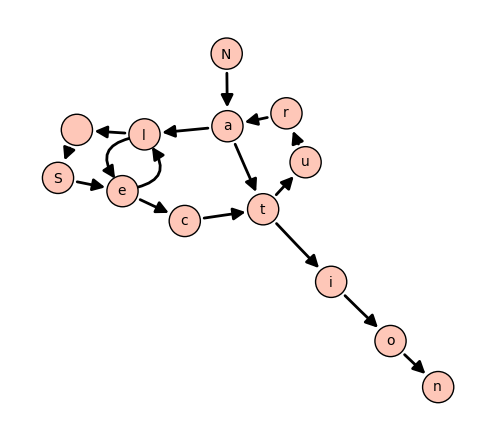Remember that uppercase characters are regarded as distinct from lowercase, and the graph loops back through any repeated characters (because my graph has one vertex per character, regardless of how often it's used). Notice also that a space counts as a character. Try entering a different phrase and see if you can follow the sequence through the graph.

WARNING: Graphing sentences is addictive. I almost couldn't stop playing with this example, typing in different things and seeing if I could trace the sequence.

Graph Database

In this example, I access a huge database of precreated graph types built into Sage:

graphs.[Tab]
(big selectable list of graph types)

graphs.DodecahedralGraph? (followed by Shift-Enter)
(detailed help for this graph type)

Now I can render the selected graph:

graphs.DodecahedralGraph().plot()3D Graph of a Graph

Let's show the prior graph example in 3D:

graphs.DodecahedralGraph().plot3d()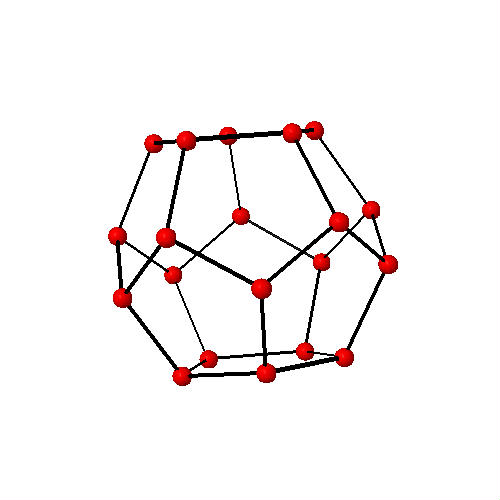When rendered by Sage, this graph can be rotated using the mouse (and the user's system must have a working Java runtime engine from http://java.com). This graph is even more impressive if you happen to have a set of 3D anaglyphic (red-cyan) glasses available — just right-click the graph and choose "Style ... Stereographic ... Red+Cyan". Then rotate the image while you view it in depth.

Here's a 3D anaglyphic teaser image for those who aren't actually running Sage (put on your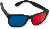3D glasses):I want to emphasize this has been just a small sampling of Sage's graphics resources — one could spend days sampling the database of graph types, as just one example.

At some point you'll want to save what you've created in Sage. The easiest way is to press the "Save" button in the notebook interface. Another useful action is to give your worksheets distinctive names. At the upper left of the notebook interface is a row of drop-down lists — choose "File ... Rename Worksheet."

If you are accessing Sage across a network and you want to make local copies of your work, choose "File ... Download to a file." This option creates a compressed copy of the current worksheet and downloads it to your machine. This local copy can later be uploaded to the Sage server for further activity, and/or archived for safekeeping.

Sage Resources
Here are some online Sage documentation resources:

The following pages go into greater depth and show how to use Sage to solve real-world problems.

Licensing"Exploring Mathematics with Sage" by Paul Lutus is licensed under a
 Home | Mathematics | * Sage | * Exploring Mathematics with Sage 02. Installation and Testing 03. Learning Sage 04. Trapezoidal Storage Tanks 05. Cylindrical Storage Tanks 06. Differential Equations 07. Future Value 08. Tuned Circuits 09. Fourier Methods 10. Polynomial Regression 11. Terminal VelocityShare This Page